Next: The Fundamental Matrix Up: No Title Previous: Homogeneous Notation Interlude

# The Essential Matrix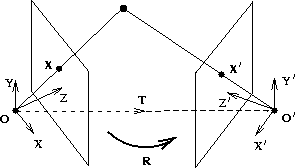There are two camera coordinate systems, related by a rotation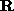and a translation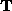: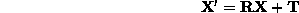Taking the vector product with, followed by the scalar product with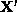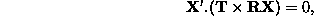which expresses that the vectors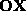,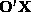and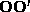are coplanar. Using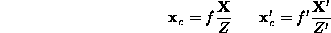gives a relation between image points in the camera coordinate systems,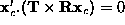. This can be written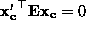, where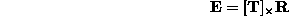is the Essential matrix. This is the algebraic representation of epipolar geometry for known calibration.

Then using the calibration matrices to relate the image point in pixels to the point in the camera coordinate system: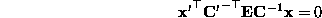which defines the Fundamental matrix,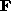by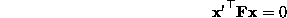where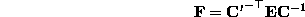Bob Fisher
Wed Apr 16 00:58:54 BST 1997